# context.fillRect()

## Overview

The `context.fillRect()` method draws a filled rectangle on the canvas by passing it four values:

``context.fillRect(x, y, width, height);``

This programs the context to draw a rectangle at (`x`, `y`) with the given `width` and `height` values. The rectangle is filled with the color, gradient, or pattern currently stored on the context's `fillStyle` property.

## Draw a Filled Rectangle

In this example, we set the context's `fillStyle` to red and then use `context.fillRect()` to draw a filled rectangle at (100, 60) with a width of 240 and a height of 180. The coordinates (100, 60) apply to the rectangle's top left corner, which is 100 pixels to the right and 60 pixels down from the origin of the context.

Change the x-coordinate, y-coordinate, width, and height of the rectangle to see what happens.

To learn more about the coordinate system and defining fill colors, visit the Coordinates and fillStyle lessons.

Quick Reference:

Message Log

## Challenge 1

Draw a red rectangle at (80, 40) with a width of 300 and a height of 200.

Message Log
##### Canvas (your drawing will display here)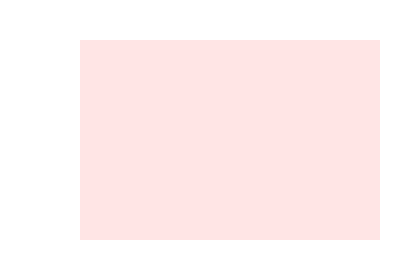## Position Rectangles Within a Shape

Positioning a rectangle is straightforward if we know the coordinates of its top left corner. But sometimes, if we have to position a rectangle relative to another rectangle, we aren't given its coordinates.

In this example, we draw the flag of Madagascar. We know the coordinates of the top left corner of the flag are supposed to be (60, 40), but what are the coordinates of the white, red, and green rectangles?

Since the top left corner of the white rectangle is the same as the top left corner of the flag, its coordinates are (60, 40).

Since the white rectangle is 100 pixels wide, the coordinates of the red rectangle are (160, 40). This positions the red rectangle 100 pixels to the right of the white rectangle.

And since the red rectangle is 100 pixels tall, the coordinates of the green rectangle are (160, 140). This positions the green rectangle 100 pixels below the red rectangle.

Quick Reference:

Message Log

## Challenge 2

Draw a larger version of the flag of Madagascar at (30, 40).

Draw the white rectangle so its width is 120 and its height is 240. Draw the red and green rectangles so their widths are 240 and their heights are 120.

Message Log
##### Canvas (your drawing will display here)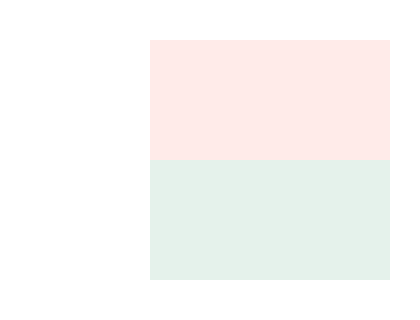## Challenge 3

Draw a dark blue rectangle directly above a dark violet rectangle.

The top left corner of the dark violet rectangle is positioned at (50, 200), and it has a width of 320 and a height of 80.

Draw the dark blue rectangle so it has the same width as the dark violet rectangle and twice the height.

Message Log
##### Canvas (your drawing will display here)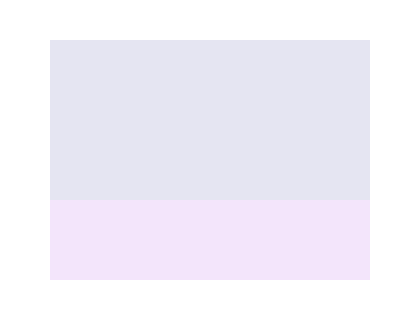## Challenge 4

Draw a green square at (140, 80) with a width and height of 120.

Then draw four smaller green squares with widths and heights of 60 touching the four corners of the larger square.

Message Log
##### Canvas (your drawing will display here)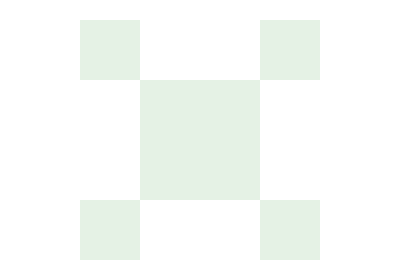## Use Draw Order to Layer Rectangles

If we program the context to draw two rectangles that overlap, the context will draw the second rectangle on top of the first rectangle.

In this example, we draw a white rectangle on top of an orange rectangle.

If we changed the order and drew the white rectangle first, then the white rectangle would be on the bottom and the orange rectangle on top.

Quick Reference:

Message Log

## Challenge 5

Draw a sky blue rectangle on top of a saddle brown rectangle.

The top left corner of the sky blue rectangle is positioned at (100, 80), and its width is 200 and its height is 160.

The saddle brown rectangle is positioned and sized so it forms a 40-pixel-wide border around the sky blue rectangle, like a picture frame.

Message Log
##### Canvas (your drawing will display here)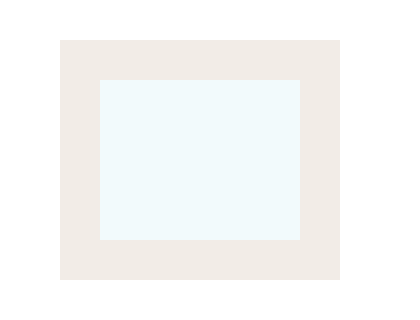## Challenge 6

Draw the image to the right.

The two plum and two lemon chiffon rectangles inside of the midnight blue border each have a width of 120 and a height of 60.

The midnight blue border is 60-pixels-wide and the top left corner of the border is positioned at (20, 20).

Note: It is possible to draw this image with only four rectangles. Can you figure out how?

Message Log
##### Canvas (your drawing will display here)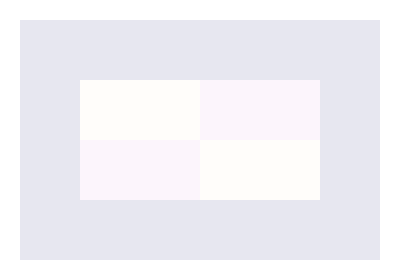## Ready for the next lesson?

Next up, the "fillStyle" lesson >